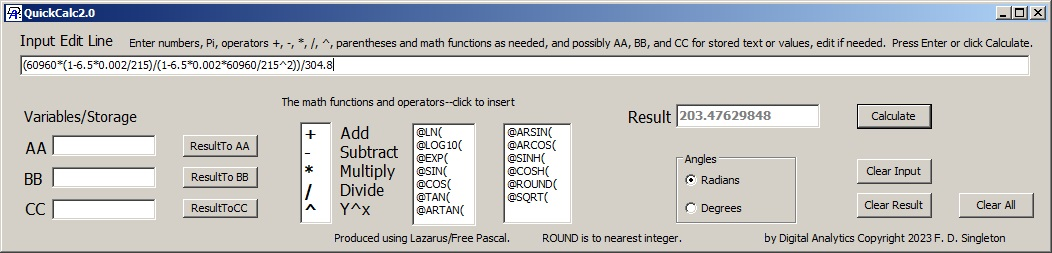Digital Analytics

## Quick Scientific Calculator

To use this calculator in the most basic way you can type an expression into the input line. When you press the "Enter" key or click on "Calculate" the calculated result appears in the Result box. If you save "Result" into boxes marked AA, BB, or CC, you can type an expression containing variables AA, BB, or CC and numbers will be substituted. (It is even possible to type fragments of expressions into AA, BB, or CC which will be substituted wherever AA or BB or CC appear in the input line. The input line and AA, BB, CC boxes all contain editable text which is translated to number values, etc, internally only after you click "Calculate" or press "Enter".)The illustration shows the calculator with a rather large formula. The same result value was obtained with an Excel formula.

There is no array of number keys because you can just as well type them on the keyboard, but there are the +,-,*,/,and ^ operators. Clicking on them inserts the operator at the blinking caret (or you can just type them). Also, a set of logarithmic and trigonometric functions are shown in two columns. Clicking inserts them. As in EDTECH they all start with @. @ARSIN( represents inverse of @SIN(, @ARCOS( inverse of @COS, etc. (Also note that while some calculators have a yx key, many just have a ^ key as here.)

There is a binary - operator as in example 5-3=2 and you can also use - as a unary operator as in -5 to negate 5 or to negate any other expression. However you can't have an expression like +5 by itself. The + is not a unary operator.

You may freely use floating point notation e.g. 1.34E-3 or 45.3e+03 etc when entering numbers. You can use pi, a number supplied by Free Pascal.

You can compute integer powers of negative numbers but with non-integer powers of negatives there is an error.

Calculations use double-precision arithmetic. If you are, say, adding up a long string of numbers, the input line can hold about 510 characters. Individual numbers can be no more than 34 characters long.